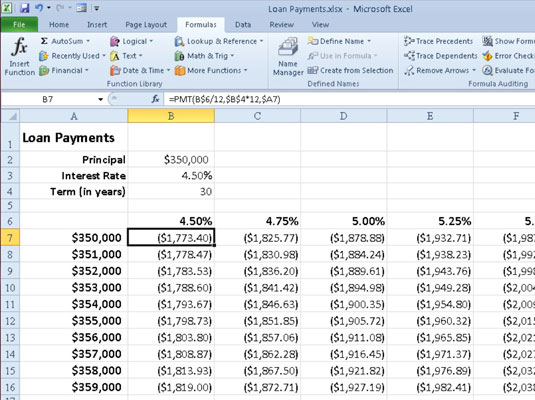##### Excel 2010 All-in-One For DummiesExcel 2010's PMT function calculates the periodic payment for an annuity, assuming a stream of equal payments and a constant rate of interest. The PMT function uses the following syntax:

`=PMT(rate,nper,pv,[fv],[type])`

As with the other common financial functions, rate is the interest rate per period, nper is the number of periods, pv is the present value or the amount the future payments are worth presently, fv is the future value or cash balance that you want after the last payment is made (Excel assumes a future value of zero when you omit this optional argument as you would when calculating loan payments), and type is the value 0 for payments made at the end of the period or the value 1 for payments made at the beginning of the period (if you omit the optional type argument, Excel assumes that the payment is made at the end of the period).

The PMT function is often used to calculate the payment for mortgage loans that have a fixed rate of interest. The following figure shows a sample worksheet that contains a table using the PMT function below to calculate loan payments for a range of interest rates (from 4.5 percent to 5.75 percent) and principals (\$350,000 to \$359,000).

`=PMT(B\$6/12,\$B\$4*12,\$A7)`

The table uses the initial principal that you enter in cell B2, copies it to cell A7, and then increases it by \$1,000 in the range A8:A16. The table uses the initial interest rate that you enter in cell B3, copies to cell B6, and then increases this initial rate by 1/4 of a percent in the range C6:G6. (The term in years in cell B4 is a constant factor that is used in the entire loan payment table. This means that every cell in range A6:G16 contains a formula except cell A6.)A Loan Payments table that uses the PMT function to calculate various loan payments.

If you create a loan table like this, you can change the beginning principal or interest rate, as well as the term, to see what the payments would be under various other scenarios. You can also turn on Manual recalculation so that you can control when the Loan Payments table is recalculated (click the Calculation Options button on the Formulas tab and select Manual).